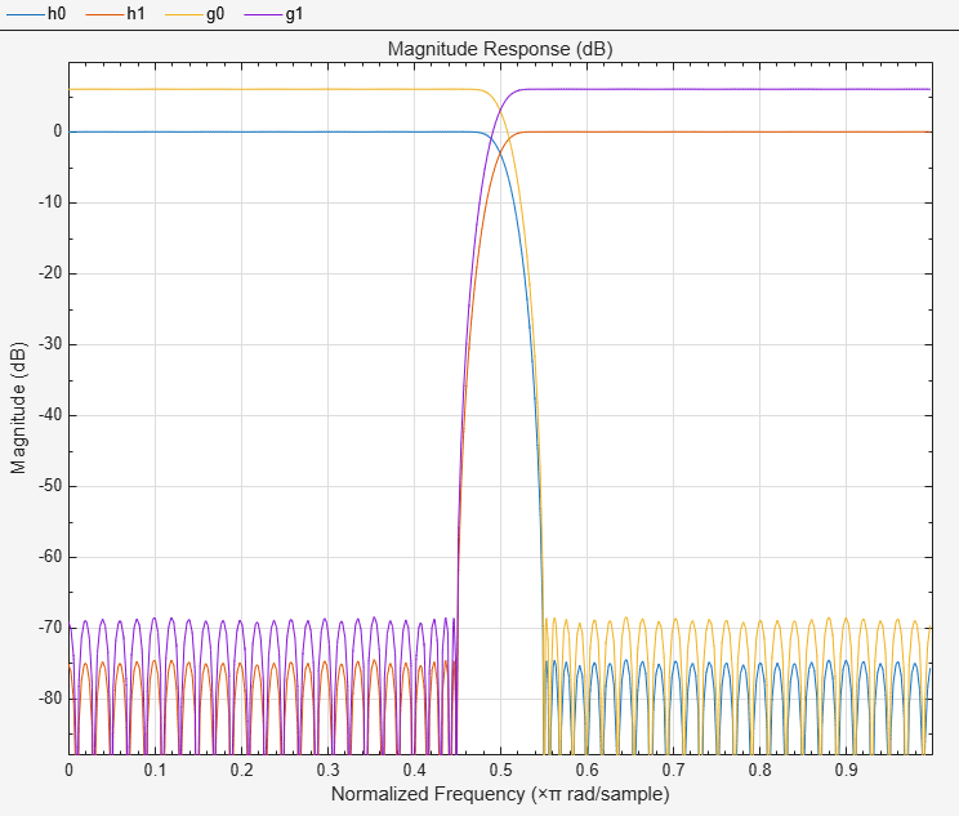# firpr2chfb

Two-channel FIR filter bank for perfect reconstruction

## Syntax

```[h0,h1,g0,g1] = firpr2chfb(n,fp) [h0,h1,g0,g1] = firpr2chfb(n,dev,'dev') [h0,h1,g0,g1] = firpr2chfb('minorder',fp,dev) ```

## Description

`[h0,h1,g0,g1] = firpr2chfb(n,fp)` designs four FIR filters for the analysis sections (`h0` and `h1`) and synthesis section is (`g0` and `g1`) of a two-channel perfect reconstruction filter bank. The design corresponds to the orthogonal filter banks also known as power-symmetric filter banks.

`n` is the order of all four filters. It must be an odd integer. `fp` is the passband-edge for the lowpass filters `h0` and `g0`. The passband-edge argument `fp` must be less than 0.5. `h1` and `g1` are highpass filters with the passband-edge given by (1-`fp`).

`[h0,h1,g0,g1] = firpr2chfb(n,dev,'dev')` designs the four filters such that the maximum stopband ripple of `h0` is given by the scalar `dev`. Specify `dev` in linear units, not decibels. The stopband-ripple of `h1` is also be given by `dev`, while the maximum stopband-ripple for both `g0` and `g1` is (2*`dev`).

`[h0,h1,g0,g1] = firpr2chfb('minorder',fp,dev)` designs the four filters such that `h0` meets the passband-edge specification `fp` and the stopband-ripple `dev` using minimum order filters to meet the specification.

## Examples

collapse all

Design a filter bank with filters of order n equal to 99 and passband edges of 0.45 and 0.55.

```n = 99; [h0,h1,g0,g1] = firpr2chfb(n,.45); fvtool(h0,1,h1,1,g0,1,g1,1); ```Here are the filters, showing clearly the passband edges.

Use the following stem plots to verify perfect reconstruction using the filter bank created by firpr2chfb.

```stem(1/2*conv(g0,h0)+1/2*conv(g1,h1)) n=0:n; stem(1/2*conv((-1).^n.*h0,g0)+1/2*conv((-1).^n.*h1,g1)) stem(1/2*conv((-1).^n.*g0,h0)+1/2*conv((-1).^n.*g1,h1)) stem(1/2*conv((-1).^n.*g0,(-1).^n.*h0)+... 1/2*conv((-1).^n.*g1,(-1).^n.*h1)) stem(conv((-1).^n.*h1,h0)-conv((-1).^n.*h0,h1)) ```## Extended Capabilities